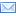#Difference Between Similar Terms and Objects

# Difference Between Dependent Variables and Independent VariablesDependent variables vs. independent variables

There are so many terms and formulas when it comes to mathematics. Some people find it fun, while other people find it very difficult to understand. But mathematics is part of life; without it science will never become a fact. Because of mathematics there is an explanation to everything; because of it life seems easier. That is why people should be eternally grateful for that day when the basic 1, 2, 3’¦ was learned. However, mathematics is not easy. It’s a total and different complexity that delves into a higher level of understanding. It’s all about the computations, the answers, or the solutions. Mathematics is a whole new language used by the most brilliants of minds.

Geometry, integers, and acute angles, are just some of the thousands of mathematical terminologies found in the glossary of the Mathematics Encyclopedia. Other terms which you should completely have to learn about and which are what this article is about are also among the thousands of math terms. These terms are similar but subtly used in different ways when it comes to math and statistics. These terms are called dependent variables and independent variables. The most important use of these two is in distinguishing two different quantities in an equation. There are certain ways in separating them and in using them until it reaches a point that the dependent variable becomes dependent to the independent variable.

These variables are very important especially when it comes to conducting experiments. This is because they can help you monitor your experiment quantitatively. By using these variables, you can properly measure your results and come up with conclusions that are very accurate.

The independent and the dependent variables are interrelated to each other. In your experiment, the independent variable is the one being changed. When the independent variable changes in your experiment, so thus the dependent variable. Also the dependent variable’s outcome depends on the independent variable. These variables are integral factor to your experiment. That is why defining and comparing them is very important.

The independent variable is the variable that the researcher manipulates in the experiment. This variable will then be hypothesized in order to influence the dependent variable. The independent variable has a big effect on the entire experiment, and is very important in drawing the conclusion of the experiment.

On the other hand, the dependent variable is the one being measured by the researcher in the experiment. It is the variable that shows how strong the effect of the independent variable is.

So, ultimately, the independent variable is the one manipulated and its effects are reflected by the dependent variable. For example, in an experiment determining how much dosage of medicine is needed to cure a certain disease, the dosage is the independent variable, while the dependent variable is whether or not the disease is cured. This is because the dosage can be changed or manipulated (you can either add or lessen the dosage). To know the effect of the independent variable, the dependent variable (which is the result if the disease is cured or not) will show the results.

SUMMARY:

1.

Independent variables are the one manipulated or changed in an experiment, while the dependent variables are the ones showing the effect or the former.
2.

The outcome of the dependent variable depends on the independent variable.
3.

Independent variable is changed then the dependent variable is measured to come up with the accurate conclusion.

### Search DifferenceBetween.net :

Custom Search

Help us improve. Rate this post!(5 votes, average: 4.80 out of 5)Loading...Email This Post : If you like this article or our site. Please spread the word. Share it with your friends/family.

### 1 Comment